Journal of Mechanical Engineering, Automation and Control Systems

Published: 21 January 2021

# The influence of the SCM structure parameters on the engine vibration and optimization the engine power

Dingao Yan1
Vanliem Nguyen2
1, 2School of Mechanical and Electrical Engineering, Hubei Polytechnic University, Huangshi, 435003, China
1, 2Hubei Key Laboratory of Intelligent Conveying Technology and Device, Hubei Polytechnic University, Huangshi, 435003, China
Corresponding Author:
Vanliem Nguyen
Views 63

#### Abstract

In this paper, based on the slider-crank-mechanism (SCM) dynamics model, the dynamic parameters of the engine including the eccentricity ($e$) between crankshaft centre and cylinder centre of the SCM, the length and radius of the connecting rod and crankshaft, and engine speed are researched on the engine vibration and power, respectively. The dynamic parameters are then optimized based on the multi-objective genetic algorithm developed in MATLAB software to improve the vibration and power of the engine. Both the values of the maximum friction force and maximum torque are chosen as the objective functions. The research results show that the length of the connecting rod ($L$), radius of the crankshaft ($R$), eccentricity $e$, and engine speed ($ne$) significantly affect the vibration and power of the engine. With the optimized dynamic parameters of $L=$ 136 mm, $R=$ 45 mm, $e=$ 14 mm, and $ne=$ 1850 rpm, the amplitude of oscillation of piston acceleration and the maximum friction force are reduced by 36.3 % and 12.5 % while the maximum torque is increased by 18.6 % in comparison without optimization. Therefore, the vibration and power of the engine are improved.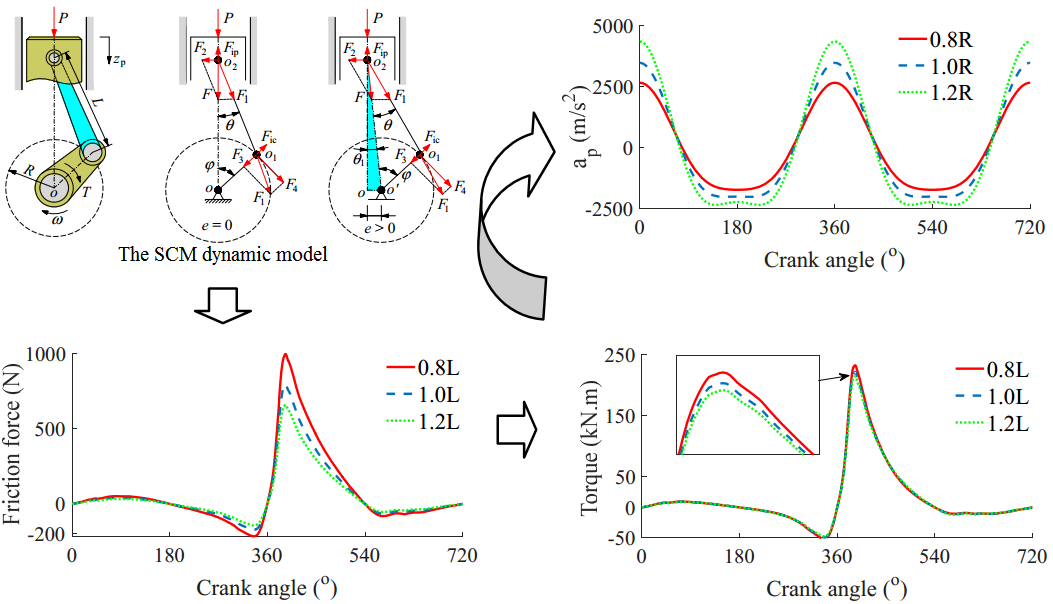#### Highlights

• The dynamic parameters of the engine including the eccentricity (e) between crankshaft centre and cylinder centre of the SCM, the length and radius of the connecting rod and crankshaft, and engine speed are researched on the engine vibration and power.
• The dynamic parameters are then optimized based on the multi-objective genetic algorithm developed in MATLAB software to improve the vibration and power of the engine.
• With the optimized dynamic parameters of L=136 mm, R=45 mm, e=14 mm, and ne=1850 rpm, the amplitude of oscillation of piston acceleration and the maximum friction force are reduced by 36.3 % and 12.5 % while the maximum torque is increased by 18.6 % in comparison without optimization.

## 1. Introduction

Nowadays, reducing engine size, increasing engine power, and reducing fuel are among the main concerns of internal combustion engine manufacturers . In order to enhance the power of internal combustion engine, the influence of the combustion chamber pressure, engine cooling system, as well as combustion mixture composition had been researched [2, 3]. Besides, intelligent fuel injection systems, variable valve timing, and engine turbochargers were developed and applied to increase the power of the internal combustion engine . The scholars reported that the efficiency of the fuel economy and improvement of the engine power were obvious. However, the levels of the vibration, noise, as well as friction power loss of the internal combustion engine were still high [5, 6]. This is also due in part to the effect of the piston skirt and washers against the cylinder borehole during the movement and relative movement of the slider crank mechanism (SCM) [7-9].

To reduce the level of the engine vibration and enhance the engine power, the horizontal impact forces between the cylinder bore and piston are researched based on the model of the SCM and the design parameters of the engine [10, 11]. Additionally, the research of the lubrication and friction problems of the piston-cylinder surfaces, crankshaft bearings, and crankpin bearings to decrease their friction power loss were also performed . Thus, the friction power loss was significantly reduced. However, the studies also noted that the dynamic loads impacting on the piston and SCM strongly affected on the increase of the vibration and friction. A optimal design of the crankpin bearing surfaces were then researched to reduce the friction power loss based on the multi-objective genetic algorithm [13, 14]. However, the studies are only interested in reducing the engine friction, the effect of the vibration has not been considered yet. Therefore, the optimization of the design parameters of the SCM may decrease the horizontal impact force to improve the vibration and friction of the engine.

In order to enhance the engine power and decrease the engine vibration, based on the SCM dynamics model, the influence of the structure parameters of the SCM such as the eccentricity between crankshaft centre and cylinder centre, length and radius of the connecting rod is evaluated. Their parameters are then optimized based on a multi-objective genetic algorithm developed in MATLAB software. The reduction of the maximum friction force and increase of the maximum engine torque are the objective functions of this study.

## 2. The dynamics model of the SCM

To estimate the influence of the SCM structure parameters on the engine vibration and optimization the engine power, the model of SCM with two cases of the eccentricities of 0 and $e>$ 0 have been illustrated for simulating and evaluating the results, as provide in Fig. 1, where, $R$, $L$, and $\omega$ are the radius of the crankshaft, the length of the connecting rod, and the angular velocity of the crankshaft, respectively.

Fig. 1The SCM dynamics model with different eccentricities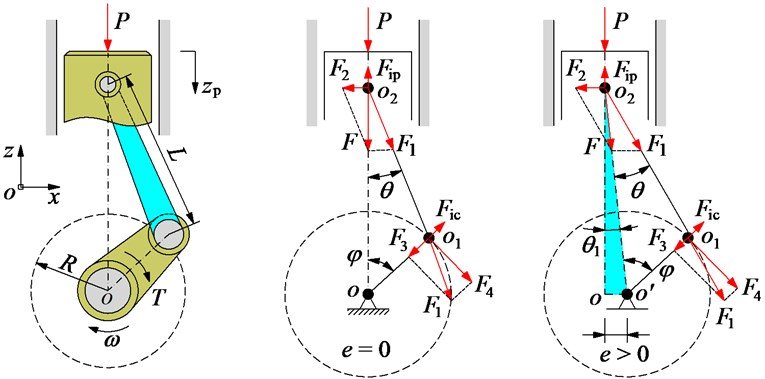Based on the model of the SCM dynamics in Fig. 1, the vibration and force equations of the SCM are then calculated by:

– Under the impact of the combustion gas pressure ($P$) on the piston peak, the displacement (${z}_{p}$) and acceleration (${a}_{p}$) equations of the piston in the $z$ direction are given by:

1
2

– The inertial force (${F}_{ip}$) of the mass of the piston-small rod end, the centrifugal inertial force (${F}_{ic}$) of the big rod end of the connecting rod, the impacting forces of $F$, ${F}_{1,2,3,4}$ of the piston affect on the connecting rod, cylinder, crankpin bearing are computed as follows:

3

Based on the dynamic equations in Eq. (3) with two different cases of the $e$, the friction force of the piston-cylinder (${F}_{f}$) and the torque of the engine ($T$) of the engine have been written by:

4

where, the friction coefficient between the piston and cylinder is defined by $\mu$.

In the existing studies of Nguyen et al. showed that the value of $\mu$ is a range of from 0.001 to 0.0015 [12, 14]. Therefore, in this study, the value of $\mu =$ 0.001 is used for evaluating the friction power loss of the engine; and the piston's acceleration, friction force, and torque of the engine $\left({a}_{p},{F}_{f},T\right)$ are the objective functions for analyzing the vibration and improving the engine power.

## 3. Simulation and result analysis

Based on the SCM parameters in Table 1 and the pressure of the engine in Fig. 2, the effect of the $L$, ${n}_{e}$, $R$, and $e$ on the vibration and power of the engine is simulated and analyzed, respectively.

Fig. 2The combustion gas pressure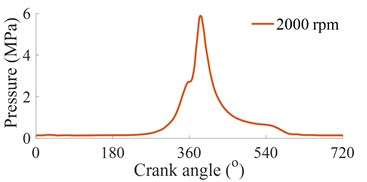Table 1The SCM operation parameters 

 Parameters Values Parameters Values $L$ / mm 129.5 ${m}_{br}$ / kg 0.250 $R$ / mm 40 $\mu$ 0.001 ${m}_{p}$ / kg 0.264 $R$ / mm 25 $m$ / kg 0.45 $\phi$ / o 0-720

### 3.1. Influence of the $\mathbit{L}$

The different length values [0.8, 1.0, 1.2]×$L$ of the connecting rod under the case of the $e=$ 0 and ${n}_{e}=$ 2000 rpm are simulated, and the simulation results are shown in Fig. 3.

The result in Fig. 3(a) (b), and (d) shows that the displacement (${z}_{p}$) and acceleration (${a}_{p}$) of the piston, and the engine torque affect on the engine’s vibration and torque are very small under the various values of $L$. However, the friction force between piston and cylinder in Fig. 3(c) significantly affect the power friction loss, especially at 0.8×$L$. Therefore, the length of the connecting rod should be chosen by {1.0 to 1.2}×$L$ to improve the engine power.

Fig. 3Influence of the L with e= 0 on the vibration and power of the engine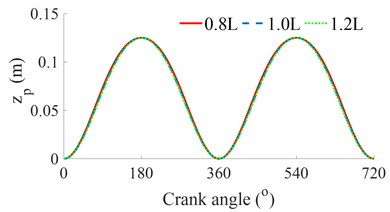a) Piston displacement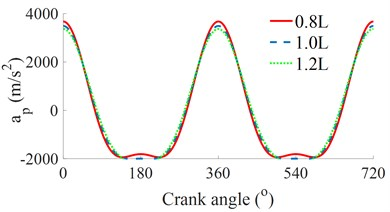b) Piston acceleration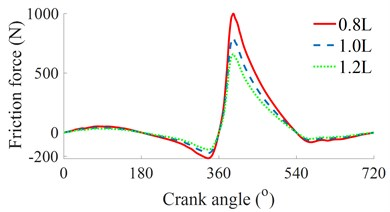c) Friction force between piston-cylinder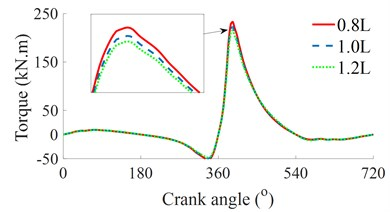d) Engine torque

### 3.2. Influence of the ${\mathbit{n}}_{\mathbit{e}}$

The different speeds of the engine [1000, 2000, 4000]×${n}_{e}$ of the connecting rod under the case of the $e=$ 0 are also simulated and analyzed. The simulation results are given in Fig. 4.

Fig. 4Influence of the ne with e= 0 on the vibration and power of the engine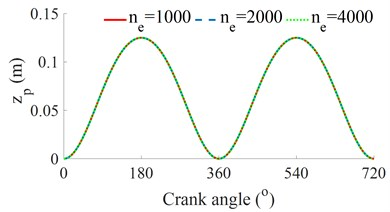a) Piston displacement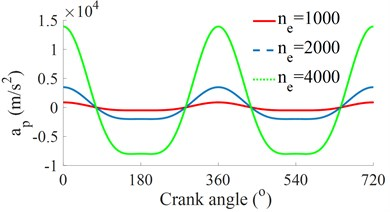b) Piston acceleration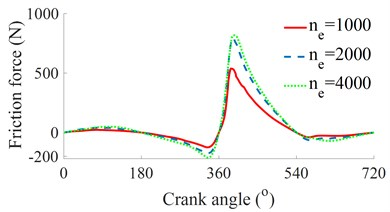c) Friction force between piston-cylinder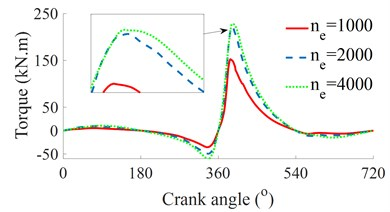d) Engine torque

The result in Fig. 4(a) of the piston’s displacement is not affected by the engine speeds. However, the piston’s acceleration, the friction force, and engine torque are strongly influenced by the engine speed, as seen in Figs. 4(b) (c), and (d). When increasing the engine speed at ${n}_{e}=$ 4000 rpm, the engine torque is significantly increased, however, both the piston acceleration and the power friction loss are also strongly increased and vice versa with ${n}_{e}=$ 1000 rpm. At the engine speed ${n}_{e}=$ 2000 rpm, the piston’s acceleration is small, but the engine’s torque is high. Therefore, the engine’s vibration and power are significantly improved at this speed. This is also the reason that the vehicle’s engines should operate at this speed. To improve the engine's vibration and power, the engine speed at {1500 to 2500}×${n}_{e}$ should be used.

### 3.3. Influence of the $\mathbit{R}$

The different values of the radius of the crankshaft [0.8, 1.0, 1.2] ×$R$ under the case of the $e=$ 0 and ${n}_{e}=$ 2000 rpm are also simulated, and the simulation results are shown in Fig. 5.

Fig. 5Influence of the R with e= 0 on the vibration and power of the engine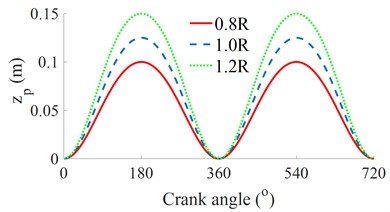a) Piston displacement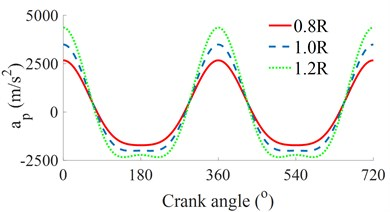b) Piston acceleration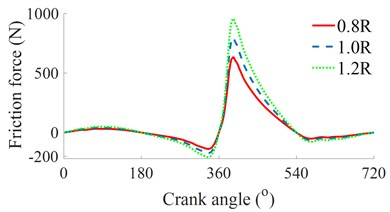c) Friction force between piston-cylinder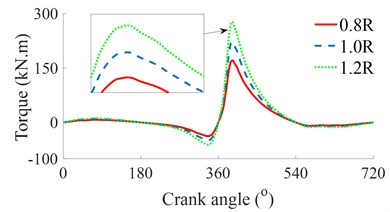d) Engine torque

All the results in Fig. 5 show that the piston’s displacement, piston’s acceleration, friction force, and torque of the engine are significantly increased with the increase of the $R$ and vice versa. Therefore, if the engine’s vibration is improved then the engine power is reduced. On the contrary, if the engine power is increased then the engine's vibration is also increased. It is to satisfy both the conditions of the reduction of the vibration and increase the power of the engine. To enhance the engine power and improve the engine vibration, the value of $R$ should be chosen by {1.0 to 1.2}×$R$.

### 3.4. Influence of the eccentricity $\mathbit{e}$

The different values of the $e$ from [0, 0.01, 0.02]×$e$ at ${n}_{e}=$ 2000 rpm are also simulated, and the simulation results are shown in Fig. 6.

The result in Fig. 6(a) shows that the piston's displacement is insignificantly affected by the $e$, however, the results in Figs. 6(b), (c), and (c) indicate that the piston’s acceleration, the friction force and the torque of the engine are remarkably affected by the $e$. All their values are increased by the increase of the $e$ and vice versa. Therefore, the improvement of the engine’s vibration and power is depended the change value of the $e$. To enhance the engine power, a range of the $e$ from {0.0 to 0.02}×$e$ should be also chosen.

Based on the above analysis results, to improve the engine power and reduce the engine vibration, the parameters of the SCM including {1.0 to 1.2}×$L\text{,}$ {1500 to 2500}×${n}_{e}\text{,}$ {1.0 to 1.2}×$R$, and {0.0 to 0.02}×$e$ need to optimize.

Fig. 6Influence of the R with e= 0 on the vibration and power of the engine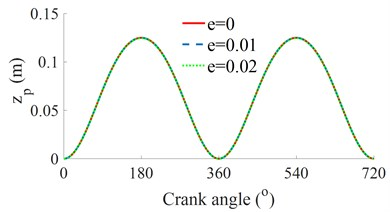a) Piston displacement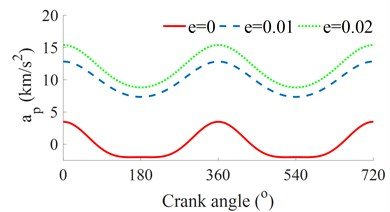b) Piston acceleration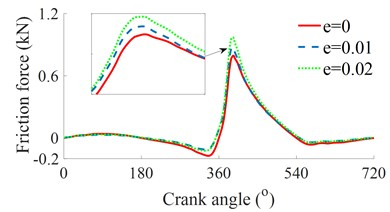c) Friction force between piston-cylinder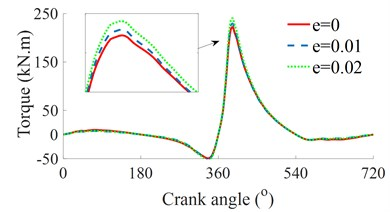d) Engine torque

## 4. Optimization of the SCM parameters

### 4.1. Application of the genetic algorithm (GA) for the optimization of SCM parameters

The GA is a multi-optimization method via the natural selection, and it is defined as finding the Maximum or Minimum functions of one or more goals. Thus, the GA is simply described by the mathematical method as follows [15, 16]:

Find the vector $a={\left[{a}_{1},{a}_{2},\dots ,{a}_{n}\right]}^{T}$ for the optimization of the function $G\left(a\right)$:

5
$G\left(a\right)=\left[{g}_{1}\left(a\right),{g}_{2}\left(a\right),...,{g}_{n}\left(a\right){\right]}^{T}.$

Subject to ${g}_{i}\left(a\right)\le$ 0, $i=$ 1, 2, ... , $p$, ${h}_{j}\left(a\right)=$ 0, $j=$ 1, 2,..., $q$, where, $G\left(a\right)$ is the vector of the study goals, and $G\left(a\right)$ must be obtained the Maximum or Minimum values. $p$ and $q$ are the boundary conditions of the optimal regions.

The GA structure has been defined by the steps of “encoding, population initialization, fitness evaluation, parent selection, genetic operations (crossover and mutate), and termination criterion”. Therefore, a flowchart of the GA program for the optimization of the vehicle dynamic parameters is plotted in Fig. 7.

The goal of this study to apply the GA is to optimize the parameters {$L$, $R$, $e$, and ${n}_{e}$} of the SCM dynamics system to reach the minimum values of the piston acceleration vibration and friction force between piston-cylinder, and the maximum value of the engine torque via the SCM dynamics model and the GA model in Figs. 1 and 7. The fitness functions of this study goal are defined as follows :

6
$\left\{\begin{array}{l}{J}_{1}=\mathrm{m}\mathrm{a}\mathrm{x}\left\{MaxT\right\},\\ {J}_{2}=\mathrm{m}\mathrm{i}\mathrm{n}\left\{Max{F}_{f}\right\}.\end{array}\right\$

Based on a program of the GA, the initial population size is established by 200, the process of genetic operations is 1000 generations, and the crossover probability and mutation probability are 0.95 and 0.05. In the optimal process of the GA, the higher value of ${J}_{1}$ and lower value of ${J}_{2}$ obtained by the GA mean that the obtained results of the parameters are the better.

Fig. 7The flowchart of the GA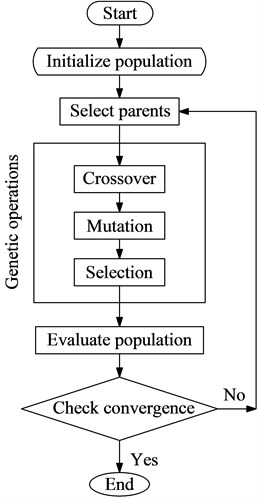Table 2The optimal region and boundary condition of the SCM dynamic parameters for the GA

 The optimal region of the dynamic parameters ${L}^{\text{'}}=$ {1.0 to 1.2}×$L$ ${R}^{\text{'}}=$ {1.0 to 1.2}×$R$ ${e}^{\text{'}}=$ {0.0 to 0.02}×$e$ ${n}_{e}^{\text{'}}=$ {1500 to 2500}×${n}_{e}$ The boundary conditions of the GA 129.5 ≤ ${L}^{\text{'}}$ ≤ 155.4 (mm) 40 ≤ ${R}^{\text{'}}$ ≤ 48 (mm) 0 ≤ ${e}^{\text{'}}$ ≤ 20 (mm) 1000 ≤ ${n}_{e}^{\text{'}}$ ≤ 6000 (rpm)

### 4.2. Optimal results and discussions

To find the optimal parameters of the SCM, based on the above analysis results, an optimal range of the SCM dynamic parameters proposed to improve the vibration and power of the engine in Table 2 are used to limit the boundary conditions of the GA. The GA is then performed to find the optimal values of ${J}_{1}$ and ${J}_{2}$ in 1000 generations.

Fig. 8The curve of fitness values of the GA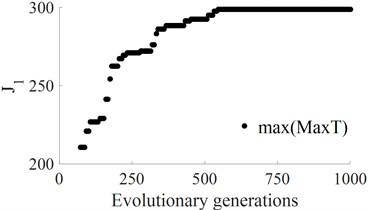a) The fitness of the maximum $T$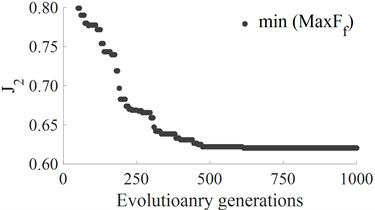b) The fitness of the maximum ${F}_{f}$

The results of the optimal running process of the ${J}_{1}$ and ${J}_{2}$ are shown in Fig. 8. The results show that the maximum fitness value of ${J}_{1}$ and the minimum fitness value of ${J}_{2}$ are reached from the value 660 of evolutionary generation to the end. Thus, the optimization of the individuals is also obtained at the value 660 of generation. By decoding, the optimized values of the SCM dynamics parameters are presented in Table 3. The optimal values of $L=$ 136 mm, $R=$ 45 mm, $e=$ 14 mm, and ${n}_{e}=$ 1850 at the generation of 660 are then simulated to evaluate the optimal performance for the improvement of the vibration and power of the engine. The simulation results are indicated in Fig. 9.

Table 3The optimal results of the SCM structure parameters

 Generation ${L}^{\text{'}}$ (mm) ${R}^{\text{'}}$ (mm) ${e}^{\text{'}}$ (mm) ${n}_{e}^{\text{'}}$ (rpm) 0 129.5 40 0 2000 1 145 46 18 5684 2 134 47 13 1445 3 155 42 11 3421 4 125 45 12 2885 5 130 43 10 2312 ... ... ... ... ... 505 133 44 11 1965 600 134 45 12 1891 650 136 44 14 1852 660 136 45 14 1850 665 136 45 14 1850 700 136 45 14 1850 ... ... ... ... ... 1000 136 45 14 1850

Fig. 9The optimal result of the SCM structure parameters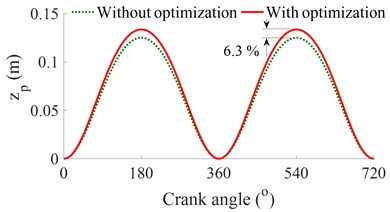a) Piston displacement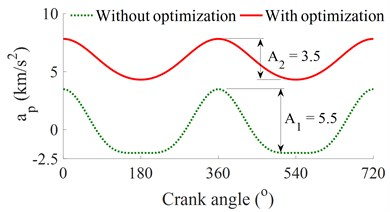b) Piston acceleration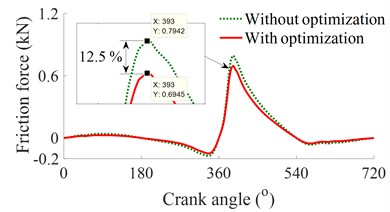c) Friction force between piston-cylinder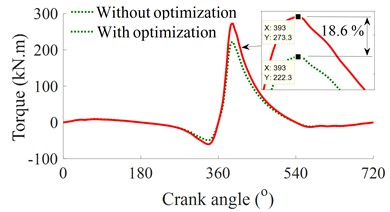d) Engine torque

The results show that the amplitude of oscillation of piston acceleration and the maximum friction force with the SCM’s parameter optimized are reduced by 36.3 % and 12.5 % while the maximum torque is increased by 18.6 % in comparison without optimization. Therefore, the vibration and power of the engine are remarkably improved.

## 5. Conclusions

The dynamic parameters of the SCM strongly affect on the engine’s vibration and power. To improve the engine power and reduce the engine vibration, the parameters of the SCM including {1.0 to 1.2}×$L$, {1500 to 2500}×${n}_{e}$, {1.0 to 1.2}×$R$, and {0.0 to 0.02}×$e$ should be concerned in the design process of the engine structure.

After the optimization of the dynamic parameters of the SCM by using the GA, the vibration amplitude of the piston’s acceleration and the maximum friction force are reduced by 36.3 % and 12.5 %, concurrently the maximum torque is increased by 18.6 % in comparison without optimization. Consequently, the vibration and power of the engine are improved.

#### Acknowledgements

This research was supported by Open Fund Project of Hubei Key Laboratory of Intelligent Transportation Technology and Device, Hubei Polytechnic University, China (No. 2020XY105) and Talent Introduction Fund Project of Hubei Polytechnic University (No. 19XJK17R).